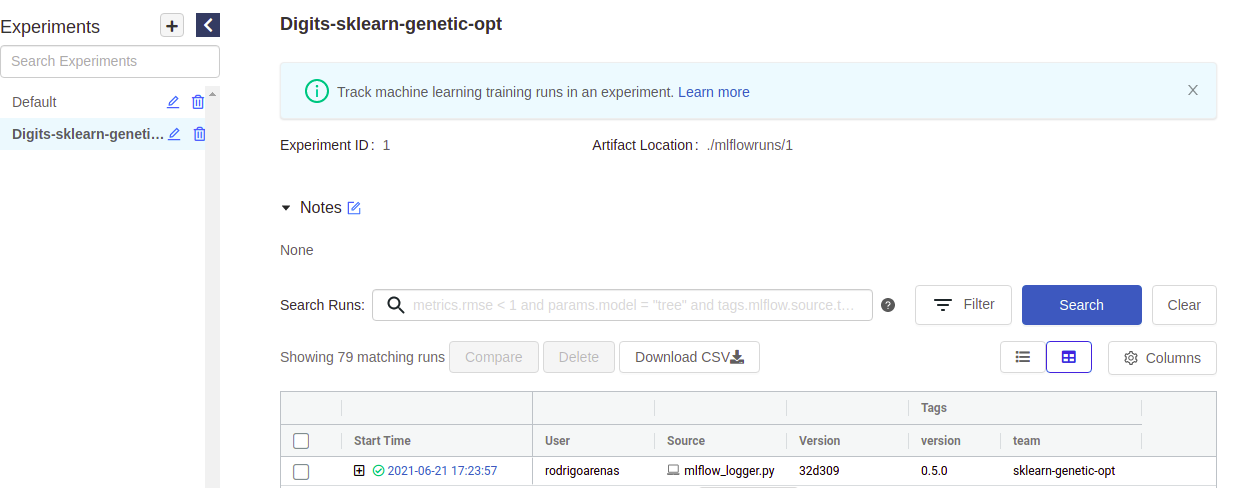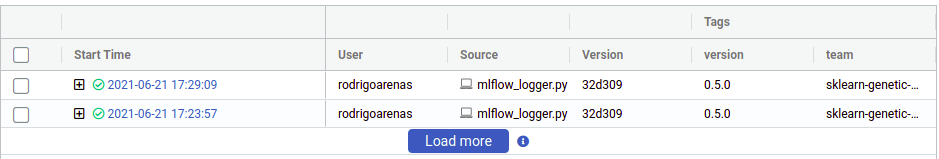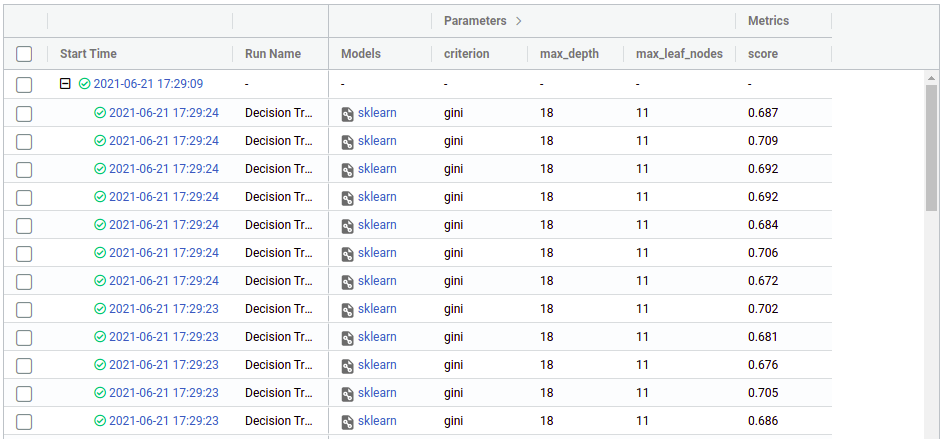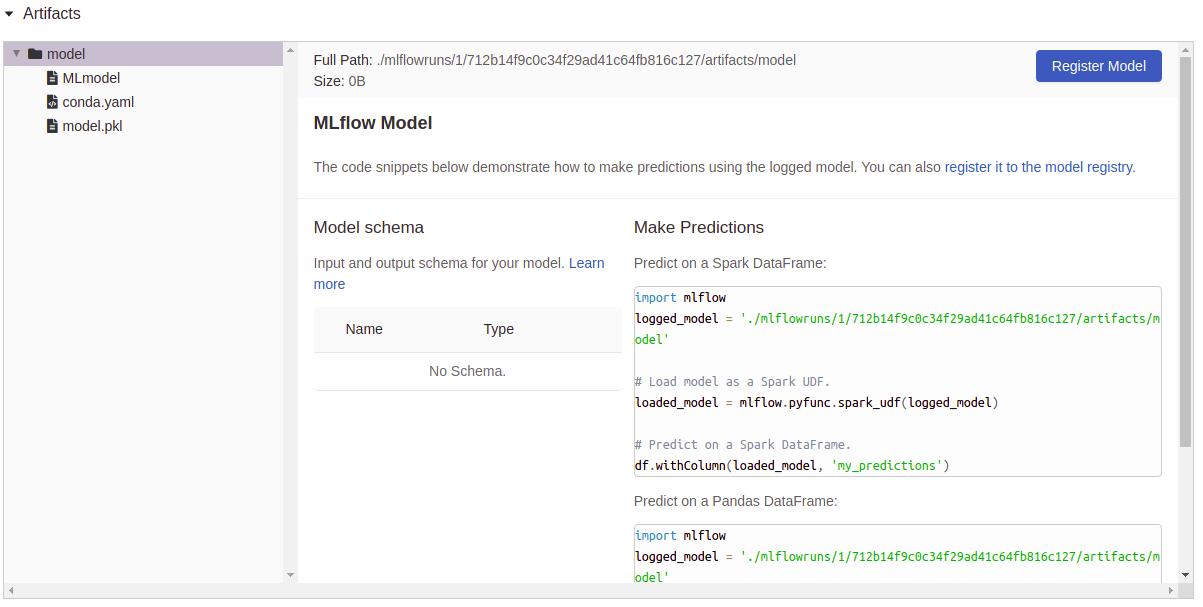# Integrating with MLflow

In this post, we are going to explain how setup the build-in integration of sklearn-genetic-opt with MLflow. To use this feature, we must set the parameters that will include the tracking server, experiment name, run name, tags and others, the full implementation is here: `MLflowConfig`

## Configuration

The configuration is pretty straightforward, we just need to import the main class and define some parameters, here there is its meaning:

• tracking_uri: Address of local or remote-tracking server.

• experiment: Case sensitive name of an experiment to be activated.

• run_name: Name of new run (stored as a mlflow.runName tag).

• save_models: If `True`, it will log the estimator into mlflow artifacts.

• registry_uri: Address of local or remote model registry server.

• tags: Dictionary of tags to apply.

## Example

In this example, we are going to log the information into a mlflow server that is running in our localhost, port 5000, we want to save each of the trained models.

```from sklearn_genetic.mlflow_log import MLflowConfig

mlflow_config = MLflowConfig(
tracking_uri="http://localhost:5000",
experiment="Digits-sklearn-genetic-opt",
run_name="Decision Tree",
save_models=True,
tags={"team": "sklearn-genetic-opt", "version": "0.5.0"})
```

Now, this config is passed to the `GASearchCV` class in the parameter named log_config, for example:

```from sklearn_genetic import GASearchCV
from sklearn_genetic.space import Categorical, Integer, Continuous
from sklearn.model_selection import train_test_split, StratifiedKFold
from sklearn.tree import DecisionTreeClassifier
from sklearn.metrics import accuracy_score
from sklearn_genetic.mlflow import MLflowConfig

label_names = data["target_names"]
y = data["target"]
X = data["data"]

X_train, X_test, y_train, y_test = train_test_split(
X, y, test_size=0.33, random_state=42)

clf = DecisionTreeClassifier()

params_grid = {
"min_weight_fraction_leaf": Continuous(0, 0.5),
"criterion": Categorical(["gini", "entropy"]),
"max_depth": Integer(2, 20),
"max_leaf_nodes": Integer(2, 30)}

cv = StratifiedKFold(n_splits=3, shuffle=True)

evolved_estimator = GASearchCV(
clf,
cv=cv,
scoring="accuracy",
population_size=3,
generations=5,
tournament_size=3,
elitism=True,
crossover_probability=0.9,
mutation_probability=0.05,
param_grid=params_grid,
algorithm="eaMuPlusLambda",
n_jobs=-1,
verbose=True,
log_config=mlflow_config)

evolved_estimator.fit(X_train, y_train)
y_predict_ga = evolved_estimator.predict(X_test)
accuracy = accuracy_score(y_test, y_predict_ga)

print(evolved_estimator.best_params_)
```

Notice that we choose small generations and population_size, just to be able to see the results without much verbosity.

If you go to your mlflow UI and click the experiment named “Digits-sklearn-genetic-opt” we should see something like this (I’ve hidden some columns to give a better look):There we can see the user that ran the experiment, the name of the file which contained the source code, our tags and other metadata. Notice that there is a “plus” symbol that will show us each of our iterations, this is because sklearn-genetic-opt will log each GASearchCV.fit() call in a nested way, think it like a parent run, and each child is one of the hyperparameters that were tested, for example, if we run the same code again, now we see two parents run:Now click on any of the “plus” symbols to see all the children, now they look like this (again edited the columns to display):From there we can see the hyperparameters and the score (cross-validation) that we got in each run, from there we can use the regular mlflow functionalities like comparing runs, download the CSV, register a model, etc. You can see more on https://mlflow.org/docs/latest/index.html

Now, as we set `save_model=True`, you can see that the column “Model” has a file attached as an artifact, if we click on one of those, we see a resume of that particular execution and some utils to use right away the model: